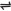## The BrØnsted-Lowry Concept of Acids And Bases

The Arrhenius concept was greatly expanded to give a more general concept of acids in the protonic definition formulated independently in 1923 by the Danish chemist J.N. BrØnsted and the English chemist T. Martin Lowry. BrØnsted and Lowry defined an acid as any molecular or ionic species that gives up a proton to another species.

### The BrØnsted-Lowry Concept of Bases

BrØnsted and Lowry defined a base as any molecular or ionic species that combines with a proton (i.e., H+). In the simplest terms, an acid is a proton donor, and a base a proton acceptor.

 HCl(aq) + NH3(g)Cl-(aq) + NH4+(aq)         (HCl is the acid, NH3 is the base)

 NH4+(aq) + H2O(l)NH3(g) + H3O+(aq)       (NH4+ is the acid, H2O is the base)

 H3O+(aq) + OH-(aq)H2O(l) + H2O(l)         (H3O+ is the acid, OH- is the base)

 H2O(l) + NH3(g)OH-(aq) + NH4+(aq)         (H2O is the acid, NH3 is the base)

Below shows a number of bases and their reactions with hydrogen ions.

 Base Reaction Acid Strength of Base
 Chloride ion, Cl- Cl- + H+HCl Very weak
 Trioxonitrate(V) ion, NO3- NO3- + H+HNO3 Very weak
 Tetraoxosulphate(VI) ion, SO42- SO42- + 2H+H2SO4 Very weak
 Ethanoate ion, CH3COO- CH3COO- + H+CH3COOH Strong
 Hydroxyl ion, OH- OH- + H+H2O Very strong

These reactions are reversible. The more the bases are able to hold onto the hydrogen ion, H+, the stronger they are, and the weaker the corresponding acids. In the same sense, the less the bases are able to hold onto the H+, the weaker they are, and the stronger their corresponding acids.

Example, the Cl- ion is a very weak base because it does not hold firmly onto the H+ to form HCl. On the contrary, HCl releases easily the H+ ion, hence, HCl is a strong acid. Hydroxide ion, OH- is a very strong base - it holds onto the H+ ion very firmly that it forms almost non-ionizable water molecule.

A substance capable of acting as a proton donor (i.e., an acid) can actually do so only in the presence of a proton acceptor (a base). Some substances are capable of acting as proton donor in the presence of stronger bases and as proton acceptor in the presence of stronger acids. Such substances are said to be amphiprotic.

Examples are H2O and the HSO4- ion. Water acts as a base when HCl dissolves in it but as an acid when the solute is NH3:

 HCl(aq) + H2O(l)Cl-(aq)+ H3O+(aq)
 H2O(l) + NH3(g)OH-(aq) + NH4+(aq)

The HSO4- ion can accept a proton from a very strong acid, and it can donate a proton to a strong base:

 H3O+(aq) + HSO4-(aq)H2O(l) + H2SO4(aq)
 HSO4-(aq) + OH-(aq)SO42-(aq) + H2O(l)

### Protolysis

All proton transfer reactions, i.e., all BrØnsted-Lowry acid-base reactions are called protolysis. The BrØnsted-Lowry concept of acids and bases applies to protolysis in any solvent. Indeed, it applies to protolysis reactions which occur when no solvent is present. The reaction between ammonia gas and hydrogen chloride gas is a BrØnsted-Lowry acid-base reaction, for a proton is transferred from the HCl molecule (the acid) to the NH3 molecule (the base):Exactly the same protolytic reaction which occurs when hydrogen chloride dissolves in liquid ammonia or in an aqueous solution of ammonia.

### Conjugate Acid and Base

According to BrØnsted-Lowry definitions, there must be a base corresponding to every acid and an acid corresponding to every base. It can be seen from the table above that each base exists corresponding to an acid.

Example, Cl- corresponds to HCl. The corresponding pairs are called conjugates, for they differ only in the presence or absence of a proton. They can be defined thus: The conjugate acid of a base is the acid which contains a proton more than the base.

Example, HCl is the conjugate acid of the base Cl- (it contains a H+, more than Cl-). The conjugate base of an acid is the base which has a proton less than the acid.

Example, Cl- is the conjugate base of the acid HCl. Restating what was said earlier: if the acid is weak, its conjugate base will be strong, and if it is strong, its conjugate base will be weak. Or, if a base is strong, its conjugate acid will be weak, and if it is weak, its conjugate acid will be strong.

Related Tutorials

 Like This Post? Please Share!!

Copyright , All Rights Reserved Free Chemistry Online | About Us | Usage of Content | Total Disclosures | Privacy Policy